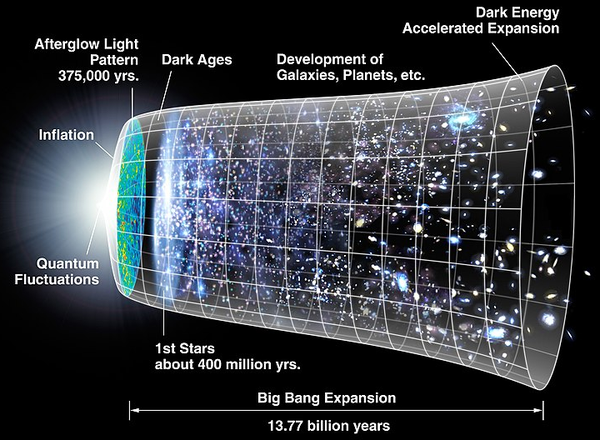Updated September 22, 2023

# Planck's wallImage: The “Planck's wall” is described as the moment from which our physical models no longer allow us to know what precedes.

## Planck's wall theory

The notion of "Planck's wall" is not commonly used in scientific literature. Instead, it is more common to speak of the "Planck length", "Planck time", and "Planck energy" as fundamental scales of physics at very high energy levels, where quantum mechanics and gravity become extreme. This theory indicates that quantum gravity becomes significant at scales close to the Planck length, calling into question the applicability of the laws of classical physics at these extreme scales. These scales are based on the fundamental constants of nature, including Planck's constant (h), the speed of light (c), and the gravitational constant (G).

- Planck's constant plays a crucial role in quantum mechanics, it is associated with the quantification of energy. It is the smallest quantity of energy that exists, i.e. 6.626 x 10^-34 joules second (J·s).
- The speed of light is a constant that intervenes in Albert Einstein's special relativity. This is the highest speed that exists, 299,792,458 meters per second (m/s).
- The gravitational constant is the constant of proportionality of Isaac Newton's universal law of gravitation. Material objects generate a gravitational force that acts on other massive objects, it is 6.67430 x 10^-11 cubic meters per kilogram per square second (m^3/kg/s^2).

According to the "Planck wall" theory, there is a characteristic length called the "Planck length" (often denoted by ℓ_P) which represents the smallest possible length scale in the universe. This Planck length is defined as:
ℓ_P = √(h * G / c^3), where G is the universal gravitational constant. Using this definition, we can derive corresponding time and energy scales, respectively the "Planck time" (t_P) and the "Planck energy" (E_P):
t_P = √(h * G / c^5),
E_P = √(c^5 * h / G).

These time and energy scales mark limits beyond which the effects of quantum gravity become dominant and where the effects of quantum mechanics cannot be neglected, even in the context of gravity. This means that when phenomena occur at energies, distances or times on the order of these Planck scales, it is necessary to take into account both quantum mechanics and general relativity to correctly describe these phenomena.

1997 © Astronoo.com − Astronomy, Astrophysics, Evolution and Ecology.
"The data available on this site may be used provided that the source is duly acknowledged."